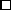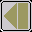### Theorem C

(Terras). (a) The set of integers with coefficient stopping time k are exactly the set of integers in those congruence classes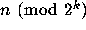for which there is an admissible vector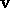of length k with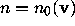.

(b) Letfor some vectorof length k. Ifis admissible, then all sufficiently large integers congruent tohave stopping time k. Ifis not admissible, then only finitely many integers congruent tohave stopping time k.

### Proof C

To prove (b), first note that ifis admissible of length k, then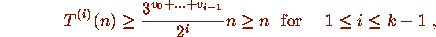and so all elements of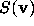have stopping time at least k. Now define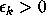by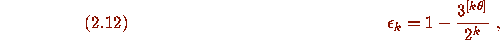where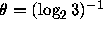, and note that (2.11) implies that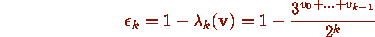for all admissible. Now for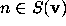for an admissible, (2.4) may be rewritten as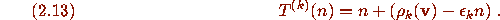Hence whenis admissible, those n inwith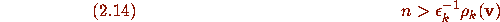have stopping time k, and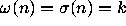in this case.

Now supposeis not admissible. There are two cases, depending on whether or not some initial segment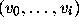ofis admissible. No initial segment ofis admissible if and only if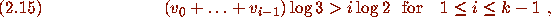and when (2.15) holds say thatis inflating. Ifis inflating,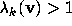so that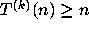for all n inby (2.4), so that no elements ofhave stopping time k or less. In the remaining casehas an initial segment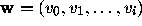with i < k -1 which is admissible. Now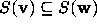and all sufficiently large elements of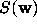have stopping time i + 1 < k by the argument just given.